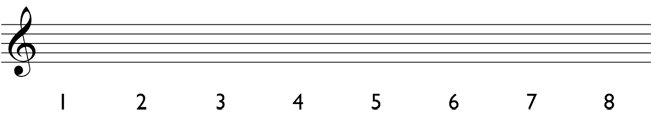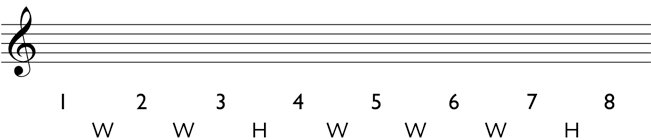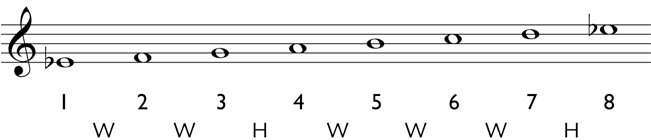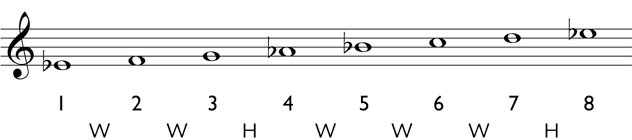# Major Scales

The major scale is one of the most common diatonic scales. Major scales consist of the following pattern of whole steps and half steps (W = whole step; H = half step).

# W W H W W W H

Here is a simple step-by-step process to follow to create major scales accurately. The E flat major scale is used as an example.

1. Write all of the scale degrees under the staff.2. Write the pattern of whole steps and half steps as they occur between each scale degree (W W H W W W H).3. Write in the pitches for that diatonic scale. Start on the note E flat and write each successive note for each scale degree. Do not skip any pitches and do not repeat any pitches. Make sure that your last note is exactly one octave above the first note of the scale. In this case, your last note should be E flat.4. Check every whole step and half step in the scale and write in the accidentals, when needed.Between scale degrees 1 and 2 is a whole step. A whole step above E flat is F, so no accidental is needed.
Between scale degrees 2 and 3 is a whole step. A whole step above F is G, so no accidental is needed.
Between scale degrees 3 and 4 is a half step. A half step above G is A flat, so you must write a flat sign in front of the A.
Between scale degrees 4 and 5 is a whole step. A whole step above A flat is B flat, so you must write a flat sign in front of the B.
Between scale degrees 5 and 6 is a whole step. A whole step above B flat is C, so no accidental is needed.
Between scale degrees 6 and 7 is a whole step. A whole step above C is D, so no accidental is needed.
Between scale degrees 7 and 8 is a half step. A half step above D is E flat, so you must write a flat sign in front of the E, to make it E flat.

Once you go through each scale degree and check the accidentals, the major scale is complete. After completing the scale, you can check the following things:
- The first and last note of the major scale should be exactly the same pitch an octave apart. For example, if your first pitch is an A flat, and your last pitch is A natural, an error has been made.
- You will never have both sharps and flats in the same major scale. For example, if you have written out a major scale and you have an F sharp and an A flat, there is an error and you should recheck your whole steps and half steps.
- In the E flat major scale above you may be tempted to write G sharp for the 4th scale degree, since it is a half step above G. However, that would be incorrect because there would be two Gs in a row, making it a non-diatonic scale. In situations like that, remember to use enharmonic equivalents. The correct note for scale degree 4 is A flat, as marked above.

Index - - Performance - Links - Kyle Coughlin
Privacy
© 2010-2018 by Kyle Coughlin and SkyLeap Music